# Physical World and Measurement Questions### (Physics) Physical World and Measurement MCQs:

Ques. Two vector A and B have equal magnitudes. Then the vector A + B is  perpendicular to
(a) A x B
(b) A – B
(c) 3A – 3B
(d) All of these

Ans. (a)

Ques. How many minimum number of coplanar vectors having different magnitudes can be added to give zero resultant?
(a) 2
(b) 3
(c) 4
(d) 5

Ans. (b)

Ques. The sum of two forces acting at a point is 16 N. If the resultant force is 8 N and its direction is perpendicular to minimum force then the forces are
(a) 6 N and 10 N
(b) 8 N and 8 N
(c) 4 N and 12 N
(d) 2 N and 14 N

Ans. (a)

Ques. A 150 m long train is moving to north at a speed of 10 m/s. A parrot flying towards south with a speed of 5 m/s crosses the train. The time taken by the parrot the cross to train would be:
(a)

30 s
(b) 15 s
(c) 8 s
(d) 10 s

Ans. (d)

Related: Plane mirror example questions

Ques. Any vector in an arbitrary direction can always be replaced by two (or three)
(a) Parallel vectors which have the original vector as their resultant
(b) Mutually perpendicular vectors which have the original vector as their resultant
(c) Arbitrary vectors which have the original vector as their resultant
(d) It is not possible to resolve a vector

Ans. (c)

Ques. A force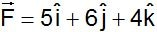acting on a body, produces a displacement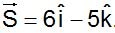Work done by the force is?

(a) 10 units
(b) 18 units
(c) 11 units
(d) 5 units

Ans. (a)

Ques. Which of the following is a vector?
(a) Pressure
(b) Surface tension
(c) Moment of inertia
(d) None of these

Ans. (d)

Ques. If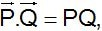then angle between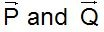is

(a) 0°
(b) 30°
(c) 45°
(d) 60°

Ans. (a)

Ques. Two cars are moving in the same direction with the same speed 30 km/hr. They are separated by a distance of 5 km, the speed of a car moving in the opposite direction if it meets these two cars at an interval of 4 minutes, will be
(a) 40 km/hr
(b) 45 km/hr
(c) 30 km/hr
(d) 15 km/hr

Ans. (b)

Ques. A particle is simultaneously acted by two forces equal to 4 N and 3 N. The net force on the particle is
(a) 7 N
(b) 5 N
(c) 1 N
(d) Between 1 N and 7 N

Ans. (d)

Ques. A boy walks uniformally along the sides of a rectangular park of size 400 m × 300 m, starting from one corner to the other corner diagonally opposite. Which of the following statement is incorrect
(a) He has travelled a distance of 700 m
(b) His displacement is 700 m
(c) His displacement is 500 m
(d) His velocity is not uniform throughout the walk

Ans. (b)

Ques. If the resultant of the two forces has a magnitude smaller than the magnitude of larger force, the two forces must be
(a) Different both in magnitude and direction
(b) Mutually perpendicular to one another
(c) Possess extremely small magnitude
(d) Point in opposite directions

Ans. (d)

Ques. If a body is in equilibrium under a set of non-collinear forces, then the minimum number of forces has to be
(a) Four
(b) Three
(c) Two
(d) Five

Ans. (b)

Ques. The vector sum of two forces is perpendicular to their vector differences. In that case, the forces
(a) are equal to each other in magnitude
(b) are not equal to each other in magnitude
(c) cannot be predicted
(d) are equal to each other

Ans. (a)

Ques. There are two force vectors, one of 5 N and other of 12 N at what angle the two vectors be added to get resultant vector of 17 N, 7 N and 13 N respectively
(a) 0°, 180° and 90°
(b) 0°, 90° and 180°
(c) 0°, 90° and 90°
(d) 180°, 0° and 90°

Ans. (a)

Ques. When three forces of 50 N, 30 N and 15 N  act on a body, then the body is
(a) At rest
(b) Moving with a uniform velocity
(c) In equilibrium
(d) Moving with an acceleration

Ans. (d)

Ques. Two equal forces (P each) act at a point inclined to each other at an angle of 120°. The magnitude of their resultant is
(a) P / 2
(b) P / 4
(c) P
(d) 2P

Ans. (c)

Ques. A person goes 10 km north and 20 km east. What will be displacement from initial point
(a) 22.36 km
(b) 2 km
(c) 5 km
(d) 20 km

Ans. (a)

Ques. Three concurrent forces of the same magnitude are in equilibrium. What is the angle between the forces ? Also name the triangle formed by the forces as sides
(a) 60° equilateral triangle
(b) 120° equilateral triangle
(c) 120°, 30°, 30° an isosceles triangle
(d) 120° an obtuse angled triangle

Ans. (a)

Related: Boolean Algebra practice quiz

Ques. The angle between the vectors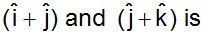(a) 30°
(b) 45°
(c) 60°
(d) 90°

Ans. (c)